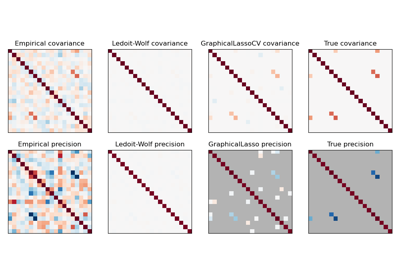# sklearn.datasets.make_sparse_spd_matrix¶

sklearn.datasets.make_sparse_spd_matrix(dim=1, *, alpha=0.95, norm_diag=False, smallest_coef=0.1, largest_coef=0.9, random_state=None)[source]

Generate a sparse symmetric definite positive matrix.

Read more in the User Guide.

Parameters
diminteger, optional (default=1)

The size of the random matrix to generate.

alphafloat between 0 and 1, optional (default=0.95)

The probability that a coefficient is zero (see notes). Larger values enforce more sparsity.

norm_diagboolean, optional (default=False)

Whether to normalize the output matrix to make the leading diagonal elements all 1

smallest_coeffloat between 0 and 1, optional (default=0.1)

The value of the smallest coefficient.

largest_coeffloat between 0 and 1, optional (default=0.9)

The value of the largest coefficient.

random_stateint, RandomState instance, default=None

Determines random number generation for dataset creation. Pass an int for reproducible output across multiple function calls. See Glossary.

Returns
precsparse matrix of shape (dim, dim)

The generated matrix.

Notes

The sparsity is actually imposed on the cholesky factor of the matrix. Thus alpha does not translate directly into the filling fraction of the matrix itself.

## Examples using sklearn.datasets.make_sparse_spd_matrix¶